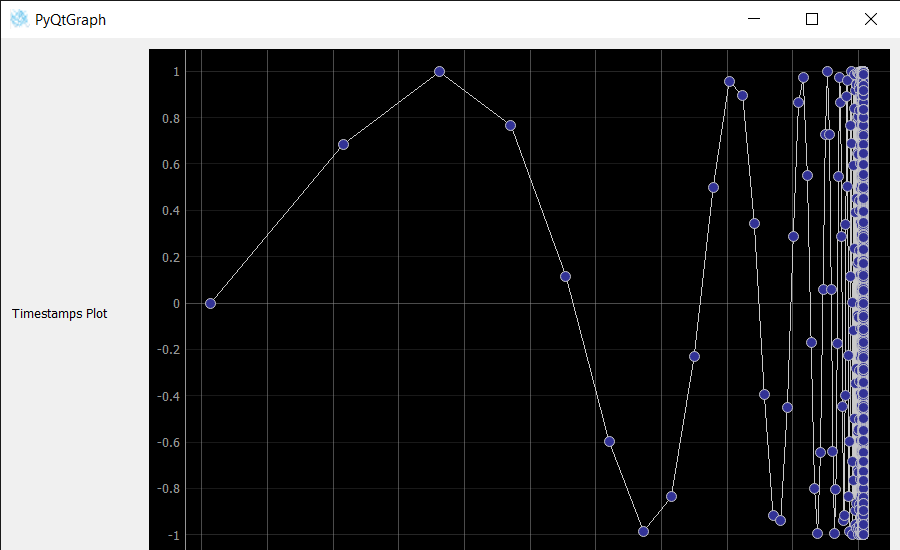Related Articles

# Plotting Data with Timestamps using PyQtGraph

• Last Updated : 31 Aug, 2021

In this article, we will see how we can plot data with timestamps using the PyQtGraph module in Python. PyQtGraph is a graphics and user interface library for Python that provides functionality commonly required in designing and science applications. Its primary goals are to provide fast, interactive graphics for displaying data (plots, video, etc.) and the second is to provide tools to aid in rapid application development (for example, property trees such as used in Qt Designer).

In order to install the PyQtGraph we use the command given below.

Attention geek! Strengthen your foundations with the Python Programming Foundation Course and learn the basics.

To begin with, your interview preparations Enhance your Data Structures concepts with the Python DS Course. And to begin with your Machine Learning Journey, join the Machine Learning - Basic Level Course

`pip install pyqtgraph`

A timestamp is a sequence of characters or encoded information identifying when a certain event occurred, usually giving date and time of day, sometimes accurate to a small fraction of a second. In this tutorial we will see how we can plot timestamps on x-axis and y-axis will have corresponding data with it.

In order to do so we have to do the following

1. Import the required libraries like pyqtgraph, pyqt5, time and numpy
2. Create a main window class using pyqt5
3. Create a graph window having axisitem set as DateAxisItem for timestamps
4. Now create a data to plot in this example we will plot the sin(1/x^2) with timestamps in the last 100 years
5. With this data plot the line graph
6. Add the graph and other widgets to the layout of central widget of main window.

Below is the implementation.

## Python3

 `# importing Qt widgets``from` `PyQt5.QtWidgets ``import` `*` `# importing system``import` `sys` `# time module``import` `time``from` `datetime ``import` `datetime, timedelta` `# importing numpy as np``import` `numpy as np` `# importing pyqtgraph as pg``import` `pyqtgraph as pg``from` `PyQt5.QtGui ``import` `*``from` `PyQt5.QtCore ``import` `*`  `class` `Window(QMainWindow):` `    ``def` `__init__(``self``):``        ``super``().__init__()` `        ``# setting title``        ``self``.setWindowTitle(``"PyQtGraph"``)` `        ``# setting geometry``        ``self``.setGeometry(``100``, ``100``, ``900``, ``550``)` `        ``# icon``        ``icon ``=` `QIcon(``"skin.png"``)` `        ``# setting icon to the window``        ``self``.setWindowIcon(icon)` `        ``# calling method``        ``self``.UiComponents()` `        ``# showing all the widgets``        ``self``.show()` `    ``# method for components``    ``def` `UiComponents(``self``):` `        ``# creating a widget object``        ``widget ``=` `QWidget()` `        ``# text``        ``text ``=` `"Timestamps Plot"` `        ``# creating a label``        ``label ``=` `QLabel(text)` `        ``# setting minimum width``        ``label.setMinimumWidth(``130``)` `        ``# making label do word wrap``        ``label.setWordWrap(``True``)` `        ``# Create a plot with a date-time axis (timestamps on x-axis)``        ``w ``=` `pg.PlotWidget(axisItems``=``{``'bottom'``: pg.DateAxisItem()})` `        ``# show the grids  on the graph``        ``w.showGrid(x``=``True``, y``=``True``)` `        ``# Plotting sin(1/x^2) with timestamps in the last 100 years``        ``now ``=` `time.time()` `        ``# x data``        ``x ``=` `np.linspace(``2` `*` `np.pi, ``1000` `*` `2` `*` `np.pi, ``8301``)` `        ``# plot the data``        ``w.plot(now ``-` `(``2` `*` `np.pi ``/` `x) ``*``*` `2` `*` `100` `*``               ``np.pi ``*` `1e7``, np.sin(x), symbol``=``'o'``)` `        ``# Creating a grid layout``        ``layout ``=` `QGridLayout()` `        ``# minimum width value of the label``        ``label.setMinimumWidth(``130``)` `        ``# setting this layout to the widget``        ``widget.setLayout(layout)` `        ``# adding label in the layout``        ``layout.addWidget(label, ``1``, ``0``)` `        ``# plot window goes on right side, spanning 3 rows``        ``layout.addWidget(w, ``0``, ``1``, ``3``, ``1``)` `        ``# setting this widget as central widget of the main window``        ``self``.setCentralWidget(widget)`  `# create pyqt5 app``App ``=` `QApplication(sys.argv)` `# create the instance of our Window``window ``=` `Window()` `# start the app``sys.exit(App.``exec``())`

Output :My Personal Notes arrow_drop_up